# Breadth-First Search Algorithm

Ever wondered how Google Maps helps you find the shortest routes within seconds? You must have encountered follow suggestions on Facebook or Instagram, wanna know-how are these social media platform that smart? Well, get on board to find solutions to all these questions.

The concept that works behind all these processes is Graph. A Graph is nothing but a non-linear data structure arranged in the format of a network of vertices or nodes and edges. A node is what holds information and an edge is the one that connects the nodes with one another.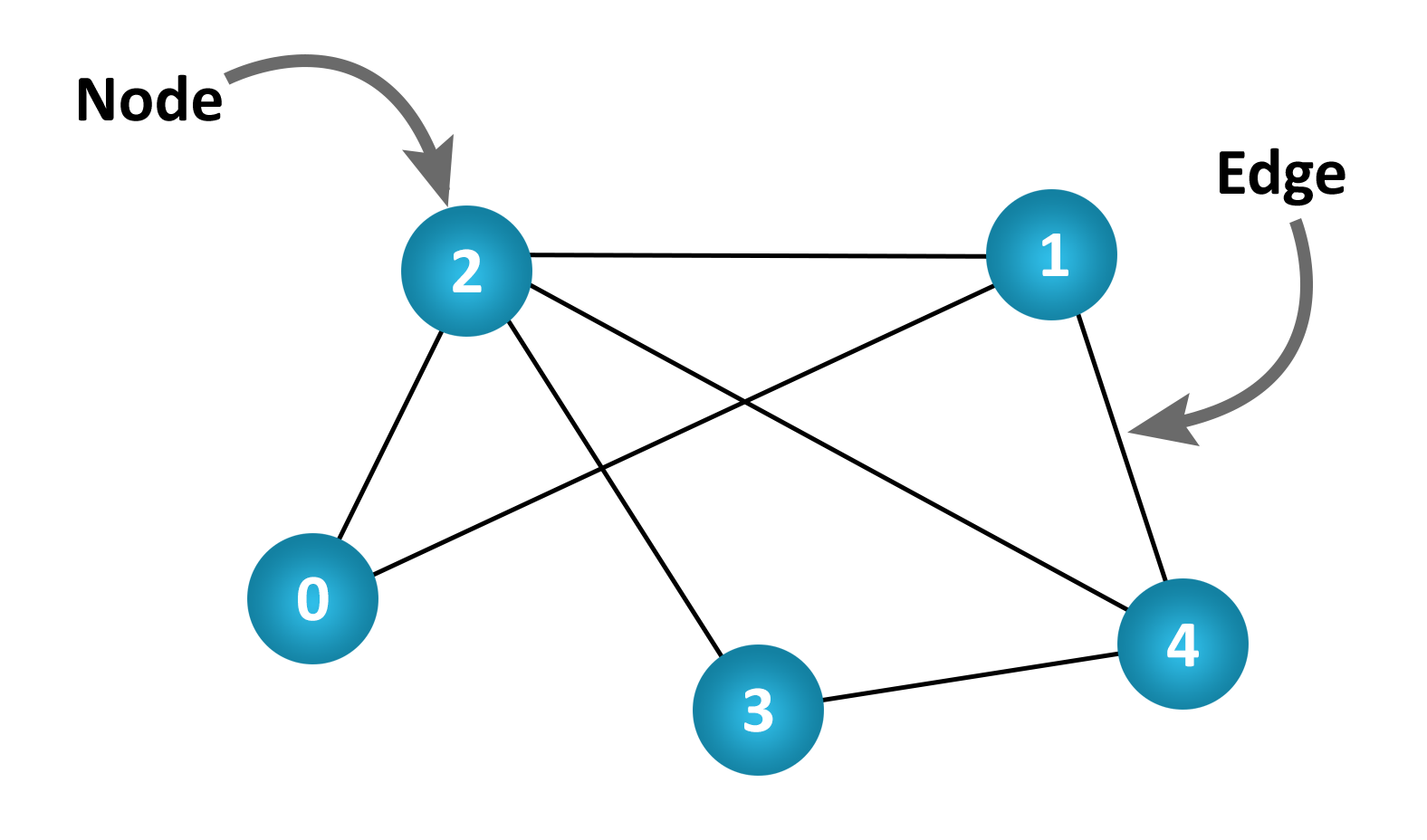Diagram-1: Nodes and Edges

The applications of Graphs are vast and are used immensely in everyday life. In Computer Science and Engineering where Computational Flow is constituted by graphs. The garbage collection of data entails BFS. The linking of web pages involves from the source web pages works on the BFS algorithm.

In view of the Internet, taking web pages as nodes and hyperlinks as edges the connection of the two webpages makes navigation through webpages possible. The World Wide Web is one of the greatest implementations of Graphs.

In the case of Social media platforms like Facebook, let us consider Facebook friends as nodes of the graph and the edge as the connection that connects them. Here, the friend suggestions employes Graphs.

There are mainly two types of categories of Graphs:
1) Directed Graphs
2) Undirected Graphs

# Directed Graphs

The category of graphs consisting of arrowheads on the edges that implies only one-way connection is called Directed Graph. The connection includes an initial and terminal node signifying that the connection is made from the initial node to the terminal node. In this case, there is a definite directed path.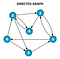Diagram-2: Directed Graphs

# Undirected Graphs

The set of graphs that have edges without arrowheads symbolizing that edge is connected to vertices on both ends are categorized as Undirected Graphs. Since both nodes are connected to one another, this category of graphs is also called Bidirectional Graph. Unlike directed graphs, they do not have initial or terminal nodes or a directed sense.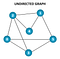Diagram-3: Undirected Graph

# Implementation of Graph

There are multiple ways to implement a data structure like a Graph. In this article, I’d be focusing on a class-based approach.

VECTOR
To begin with, create a class Vector. It is a data structure that implements a dynamic array. Vector possesses the competency to modify its dimensions as per need which makes it worthwhile. It is the Vector that contributes to the efficiency and structure of the program.

GRAPH
Make a class called Graph. Since Graph consists of two major components Nodes and Edges, a private sub-class Node would be constructed inside of the existing class Graph. The nodes would be stored in Vector so that graph can hold numerous nodes without any limitation (except for when the system runs out of memory). Having this done, we are left with establishing connections between Nodes which is done by a function connect of the graph. Apart from storing data, every Node object puts down the neighbouring elements in its own vector that makes the connections running in the Graph. The connect function performs this task of adding neighbours to the Node which gets stored in the Vector of every Node. This altogether forms connections between nodes.

Breadth-First Search
Breath-First Search is an algorithm that revolves around traversing through a data structure. In the case of graphs, BFS entails traversing through all the nodes exactly once.

For enforcing Breadth-First Search, there are two obligations to be followed, one, keeping a record of which node has been visited, and two maintaining the current node. Former concerns the implementation of a boolean array that turns the index value true every time an element is visited. Latter requires carrying through a queue. The queue upholds the current element and pops it before pushing the neighbouring element of the current node into the queue. The algorithm can be applied to both Directed and Undirected graphs provided the connections are made correspondingly.

# APPROACH

To execute BFS let’s commence by making a function that would require the start node and result queue (that would store the sequence to be printed at the end) as parameters.
The start node is pushed into the Queue and is marked visited in the Visited Array.

Run a loop that would execute until the queue is empty. Inside the loop, pop the first pushed element and assign it as the current node which gets stored in a separate queue that stores the result (i.e. the second parameter of the function). Now the neighbouring elements of the current node are checked for whether or not they have been visited, if not then they are pushed into the queue and marked visited in the array. Once the loop runs for the first time, the queue is checked for being empty this process goes on for every node.
At last, the resultant queue is printed giving us the Shortest path to traverse through nodes of the Graph.

# ALGORITHM

The breadth-first search seems really simple once you’re through all the steps that follow. All the nodes are to be visited exactly once, not less, not more.
Let’s take an illustration for your better understanding.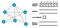Diagram-4: ILLUSTRATION

Here a graph has been made with some number written on the nodes for identification. You can see an empty queue. Only when an element is found to be not been visited then it is pushed in the queue. All the elements of the boolean array are initialized as zero. This symbolizes that none of the nodes have been visited so far.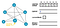Diagram-5: starting node pushed

Assuming node-2 as the starting node, we push node-2 in the queue and mark the corresponding boolean value as true/ 1.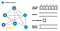Diagram-6: Look for neighbours of node-2

The first pushed element is popped from the queue and the currentNode variable is assigned as 2. All the values of currentNode are stored in the result queue to be printed at the end. Thus, node-2 is stored in the result queue. There are 3 nodes connected directly with node-2, they are node-0, node-3 and node-4.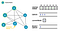Diagram-7: Check if the neighbours have been visited

Since none of the neighbours of node-2 have been visited even once, they can be pushed. Thus node-0, node-3 and node-4 are pushed in the queue. The pushed nodes are marked visited in the visited array.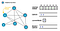Diagram-8: reassign currentNode

The first pushed element among the present elements from the queue is popped, i.e. node-0 is popped and assigned as the current node. The corresponding index of node-0 is marked true so that we do not reconsider for graph traversal. Node-0 is pushed in the result queue. The neighbouring elements of the node-0 are checked whether or not they have been visited or not.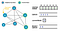Diagram-9: pushing the neighbours

Node-1, node-2, node-3 and node-5 are the neighbours of node-0. Since node-3 and node-2 have been visited before, thus, they will not be pushed in the queue. However, node-3 and node-1 and node-5 would be pushed in the queue. The pushed nodes will be marked visited in the array.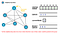Diagram-10: move to the next currentNode

Node-3 is popped from the queue, is assigned as the currentNode, and inserted in the result queue. The neighbouring elements of node-3 are node-0, node-2, node-5, node-7 that are taken for analyzing whether or not these elements’ corresponding index value is 0. Among all the directly attached elements from node-3, all the elements have been visited. Therefore, none of the elements would be considered for pushing in the queue.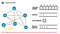Diagram-11: pop the element from the queue and assign it as currentNode

When Node-4 becomes the currentNode the directly attached elements node-1 and node-2 are found to be visited. Thus none of these nodes is pushed in the queue.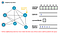Diagram-13: Nodes not pushed

Similarly, in the case of currentNode = 1, where neighbouring nodes are node-0, node-3 and node-4. Every directly linked node has been traversed once and would not be visited again, according to the algorithm.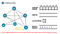Diagram-14: Nodes not pushed

Just like the previous case, when currentNode = 5, the neighbouring nodes i.e. node-0, node-1 and node-3 are found to be marked visited in the array, thus none of these are pushed in the array.
Since node-5 is the last node.
Once node-5 is popped, the queue becomes empty and graph traversal comes to an end.

The output of the program is as follows: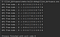Figure-1: Output

The basic logic that works here is keeping track of visited elements and implementing the queue in a way that each node is checked for its neighbouring elements until the queue is found empty.

Some of the many applications of Breadth-First Search are, finding adjacent nodes in the scenario of peer-to-peer networks, finding the nearest locations using GPS Navigation, broadcasting data in Communication Networks.